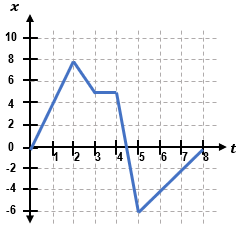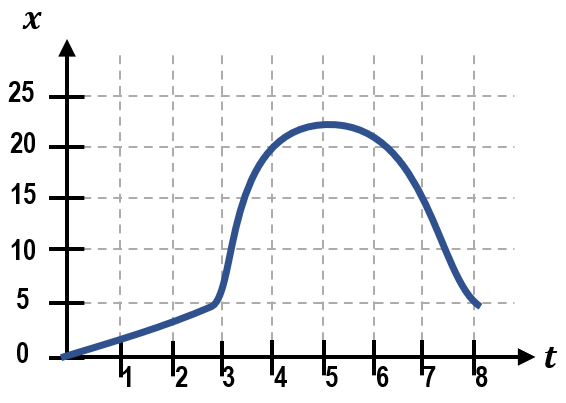Clutch Prep is now a part of Pearson
Ch 02: 1D Motion / KinematicsWorksheetSee all chapters

# Position-Time Graphs & Velocity

See all sections

Concept #1: Intro to Position-Time Graphs & Velocity

Practice: The position-time graph for a moving box is shown below.(a) What is the box’s average velocity from 0 to 5s?
(b) What is the box’s average velocity from 0 to 8s?
(c) What is the box’s velocity in the interval where it’s moving fastest?

Concept #2: Curved Position-Time Graphs & Acceleration

Concept #3: Position-Time Graphs and Instantaneous Velocity

Practice: The position-time graph for a ball on a track is shown below.
(a) What is the ball’s velocity at 4s?
(b) At what time(s) is the ball approximately travelling at -10m/s?
(c) From t = 3 to 7s, what is the sign of the acceleration?